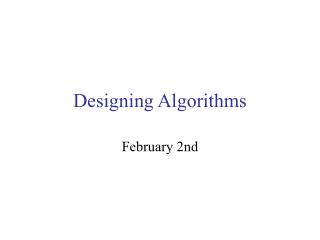DownloadDownload PresentationDesigning Algorithms

# Designing Algorithms

Télécharger la présentation## Designing Algorithms

- - - - - - - - - - - - - - - - - - - - - - - - - - - E N D - - - - - - - - - - - - - - - - - - - - - - - - - - -
##### Presentation Transcript

1. Designing Algorithms February 2nd

2. Administrativia • Lab assignments will be due every Monday • Lab access • Searles 128: daily until 4pm unless class in progress • Searles 117: 6-10pm, Sat-Sun 12-10pm • Office hours: • Mon, Wed 1-2, Tue 2:30-3:30, Thu 4-5pm • Anytime I am in the office • Email to set up appointment

3. Designing algorithms • Last time • Pseudocode • Algorithm 1: adding 2 m-digit numbers • Algorithm 2: computing miles-per-gallon • Today • More algorithms • Reading: chapter 2

4. An example pseudocode algorithm (Fig 1.2) • Given m ≥ 1 and two positive numbers a and b, each containing m digits, compute the sum c = a + b. • 0 Get values for m, am-1 … a0 and bm-1 … b0 • 1 Set the value of carry to 0. • 2 Set the value of i to 0. • 3 Repeat steps 4-6 until i > m-1 4 Set the value of ci to ai + bi + carry 5 if ci ≥ 10 then • subtract 10 from ci and set the value of carry to 1 else set the value of carry to 0 6 Add 1 to i • 7 Set the value of cm to carry • Print value of c = cm cm-1 cm-2 … c0 • Stop

5. The final MPG program (Fig 2.5) • Write a pseudocode algorithm to compute the distance travelled and the average miles per gallon on a trip when given as input the number of gallons used and the starting and ending mileage readings on the odometer. • 0 Set response to “Yes” 1 Repeat steps 2-8 until response = “No” 2 Get gallons, start, end 3 Set distance to end - start 4 Set mpg to distance ÷ gallons 5 Print mpg 6 if mpg > 25.0 then print “You are getting good gas mileage” else print “You are NOT getting good gas mileage” 7 Print “Do you want to do this again, Yes or No?” 8 Get response 9 Stop

6. Designing Algorithms: A Methodology Read the problem, identifying the input and the output. What variables are needed? What computations are required to achieve the output? Usually, the first steps in your algorithm bring input values to the variables. Usually, the last steps display the output So, the middle steps will do the computation. If the process is to be repeated, add a loop around it.

7. This visual model is good for design, too… Computer Algorithm Input (keyboard) Output (screen) Variables

8. How was the MPG program (Fig 2.5) designed? • Problem statement (p 35): • Write a pseudocode algorithm to compute the distance travelled and the average miles per gallon on a trip when given as input the number of gallons used and the starting and ending mileage readings on the odometer. Input: number of gallons, starting mileage, ending mileage Output: distance traveled, average miles per gallon Variables: gallons, start, end, distance, mpg Calculate: distance = end - start mpg = distance / gallons Put the steps in order: input, calculate, output (steps 2-8) Determine if a loop is needed (steps 0, 1, 9, 10)

9. Visualizing the program design Computer Input (keyboard) Output (screen) 25 12000 13000 response Yes gallons start end distance mpg

10. Designing a Sequential Search Algorithm • Problem statement (p 43-44): Write a pseudocode algorithm to find the location of a target value in a list of values. Input: a list of values and the target value Output: the location of the target value, or else a message that the value does not appear in the list. Variables: target, list, found, index Calculate: if the value at index in list = target set found to true else increment the index by 1 Put the steps in order: input, calculate, output Determine if a loop is needed

11. The final sequential search program (Fig 2.9) Get the value of target, n, and the listof n values Set index to 1 Set found to false Repeat until found = true orindex > n If the value of listindex = target then Output the index Set found to true else Increment the index by 1 If not found then Output a message that target was not found Stop

12. Variations of sequential search.. • Modify the sequential search algorithm such that • To find all occurrences of target in the list and print the positions where they occur • To count the number of occurrences of target in the list • To count how many elements in the list are larger than target

13. More algorithms • Write algorithms to find • the largest number in a list of numbers (and the position where it occurs) • the smallest number in a list of numbers (and the position where it occurs) • the range of a list of numbers • Range= largest - smallest • the average of a list of numbers • the sum of a list of numbers

14. For next time.. • Think about the problems • Read Chapter 2 • Except 2.3.3 (Pattern matching), which we’ll do next time